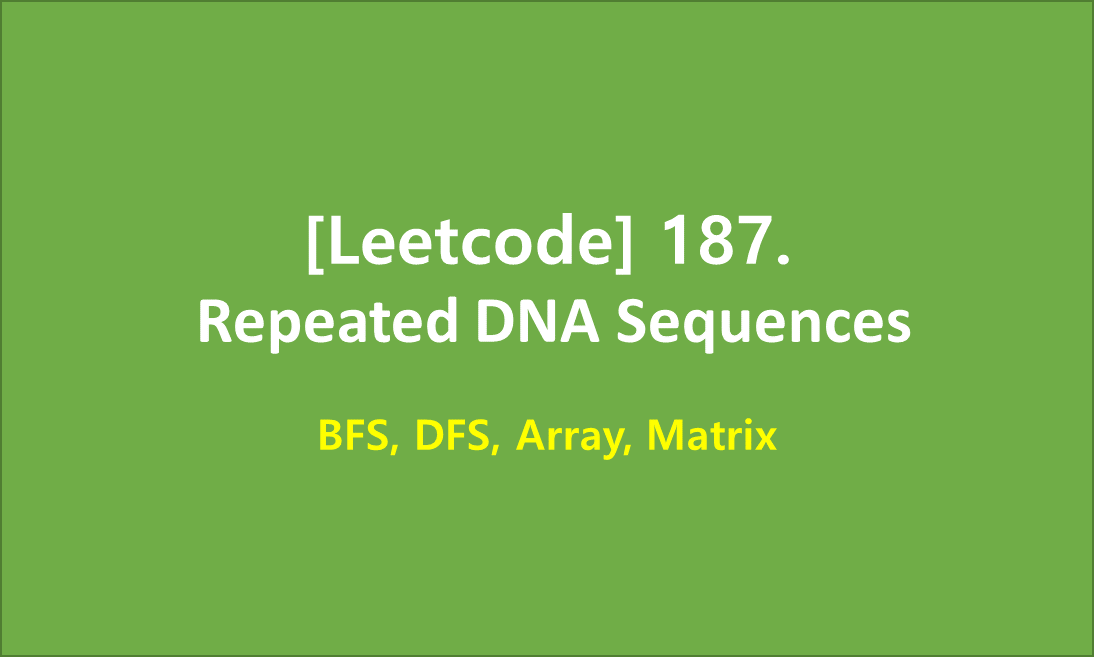# [Leetcode]187. Repeated DNA Sequences

limelimejiwon·2022년 5월 19일
0

## Python으로 공부하는 코딩테스트

목록 보기
64/67## 📄 Description

The DNA sequence is composed of a series of nucleotides abbreviated as 'A', 'C', 'G', and 'T'.

For example, "ACGAATTCCG" is a DNA sequence.
When studying DNA, it is useful to identify repeated sequences within the DNA.

Given a string s that represents a DNA sequence, return all the 10-letter-long sequences (substrings) that occur more than once in a DNA molecule. You may return the answer in any order.

### Example 1:

Input: s = "AAAAACCCCCAAAAACCCCCCAAAAAGGGTTT"
Output: ["AAAAACCCCC","CCCCCAAAAA"]

### Example 2:

Input: s = "AAAAAAAAAAAAA"
Output: ["AAAAAAAAAA"]

### Constraints:

• 1 <= s.length <= 105
• s[i] is either 'A', 'C', 'G', or 'T'.

## 💻 My Submission

class Solution:
def findRepeatedDnaSequences(self, s: str) -> List[str]:

_dict=defaultdict(int)

for i in range(len(s)-9):
_dict[s[i:i+10]]+=1

return [x for x in _dict if _dict[x]>1]

## 🕐 Complexity

Time Complexity: O(n)
Space Complexity: O(1)

References